# N. Bilic - Supersymmetric Dark Energy

Sep. 11, 2011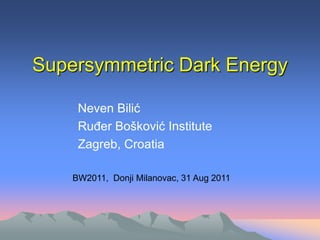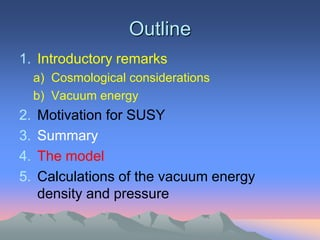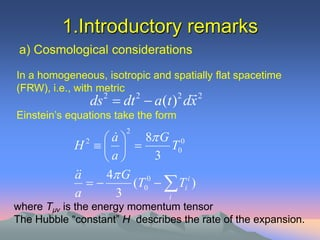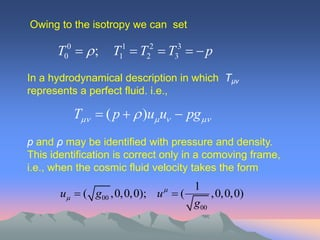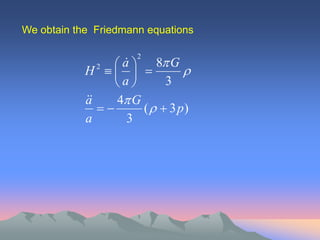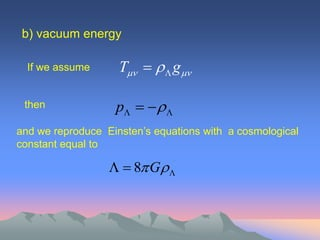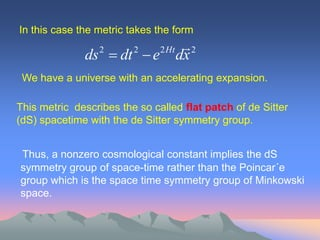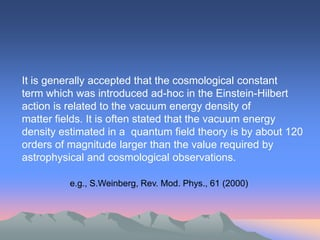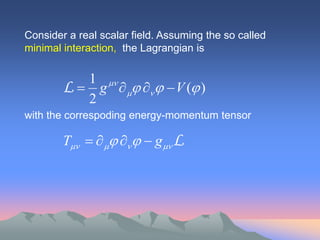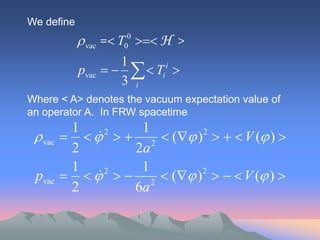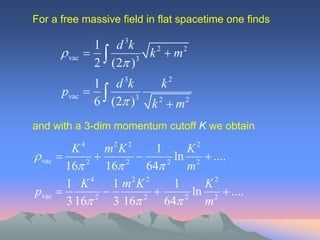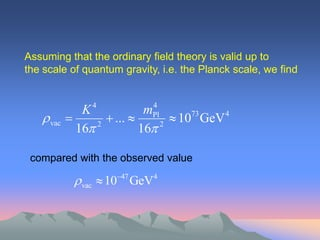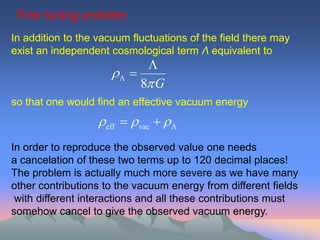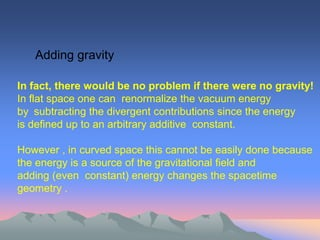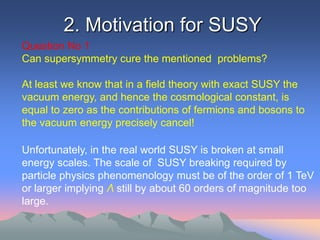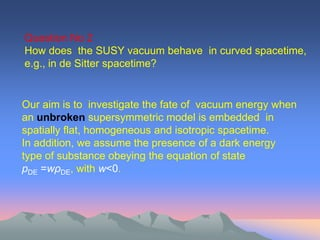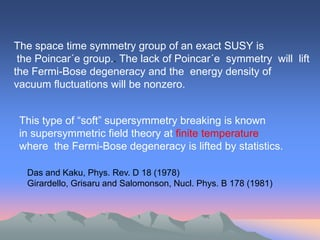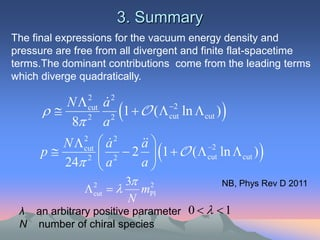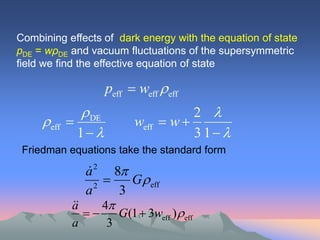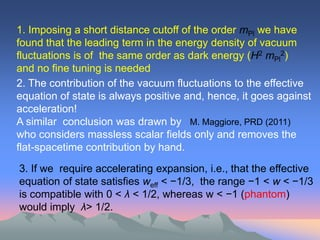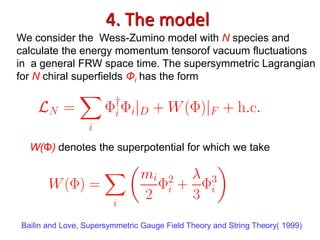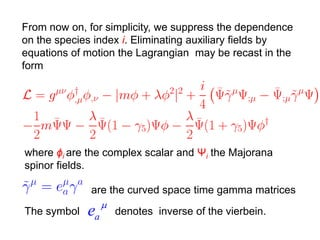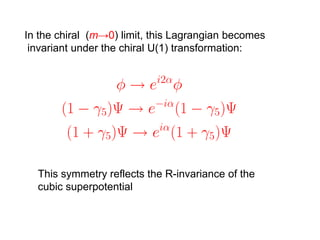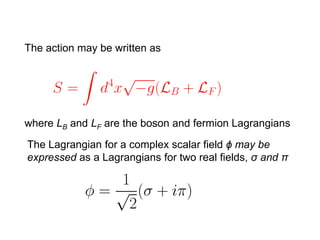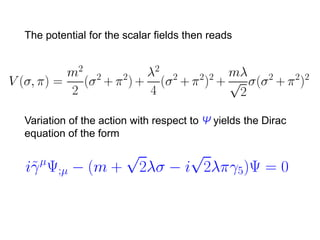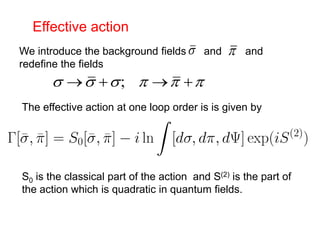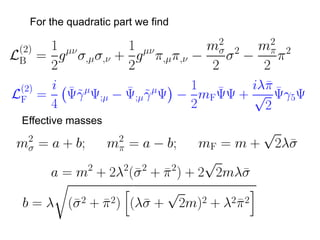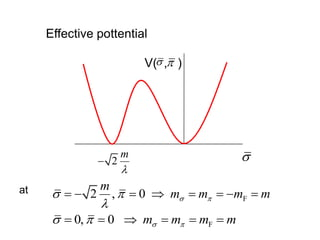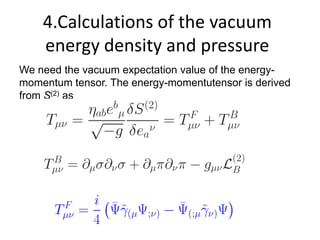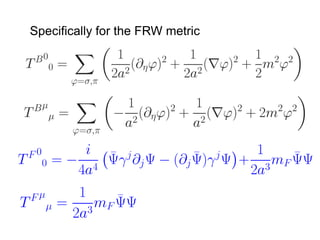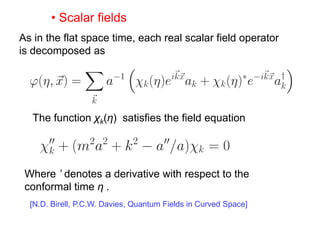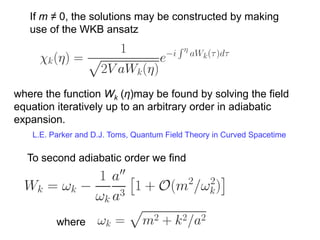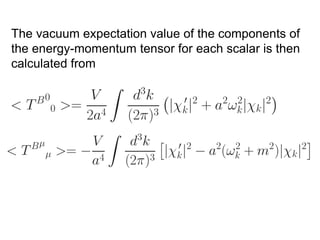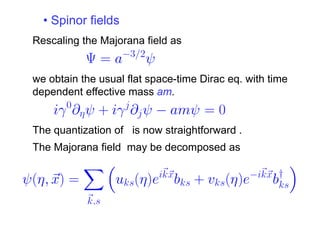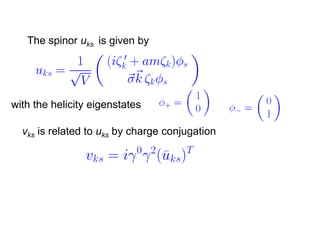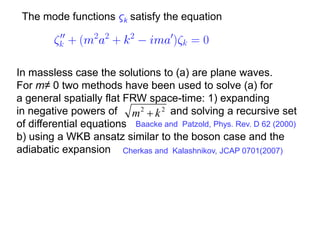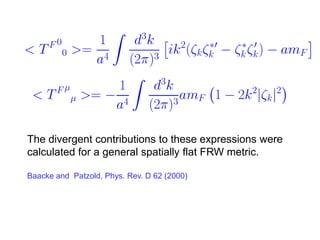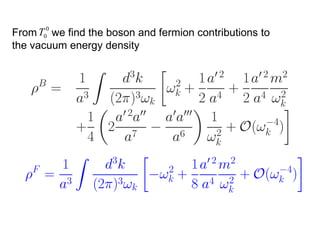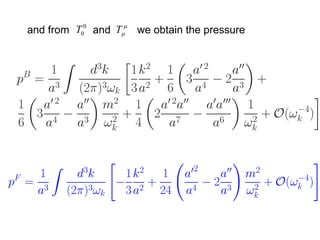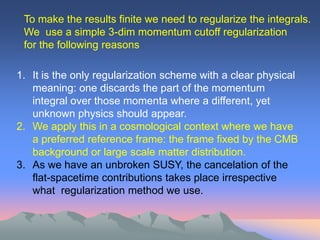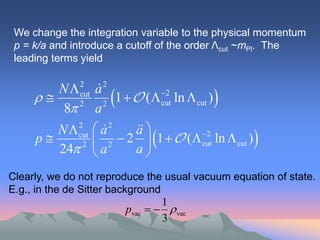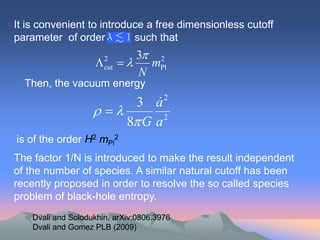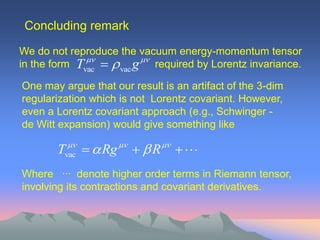1 of 45

### N. Bilic - Supersymmetric Dark Energy

• 1. Supersymmetric Dark Energy Neven Bilić Ruđer Bošković Institute Zagreb, Croatia BW2011, Donji Milanovac, 31 Aug 2011
• 2. Outline 1. Introductory remarks a) Cosmological considerations b) Vacuum energy 2. Motivation for SUSY 3. Summary 4. The model 5. Calculations of the vacuum energy density and pressure
• 3. 1.Introductory remarks a) Cosmological considerations In a homogeneous, isotropic and spatially flat spacetime (FRW), i.e., with metric  ds  dt  a(t ) dx 2 2 2 2 Einstein’s equations take the form  a  8 G 0 2  H    2 T0 a 3  a 4 G 0  (T0   Ti ) i a 3 i where Tµν is the energy momentum tensor The Hubble “constant” H describes the rate of the expansion.
• 4. Owing to the isotropy we can set T00   ; T11  T22  T33   p In a hydrodynamical description in which Tµν represents a perfect fluid. i.e., T  ( p   )u u  pg p and ρ may be identified with pressure and density. This identification is correct only in a comoving frame, i.e., when the cosmic fluid velocity takes the form  1 u  ( g00 ,0,0,0); u  ( ,0,0,0) g00
• 5. We obtain the Friedmann equations  a  8 G 2  H    2  a 3  a 4 G  (   3 p) a 3
• 6. b) vacuum energy If we assume T    g  then p     and we reproduce Einsten’s equations with a cosmological constant equal to   8 G 
• 7. In this case the metric takes the form 2 ds  dt  e 2 2 2 Ht dx We have a universe with an accelerating expansion. This metric describes the so called flat patch of de Sitter (dS) spacetime with the de Sitter symmetry group. Thus, a nonzero cosmological constant implies the dS symmetry group of space-time rather than the Poincar´e group which is the space time symmetry group of Minkowski space.
• 8. It is generally accepted that the cosmological constant term which was introduced ad-hoc in the Einstein-Hilbert action is related to the vacuum energy density of matter fields. It is often stated that the vacuum energy density estimated in a quantum field theory is by about 120 orders of magnitude larger than the value required by astrophysical and cosmological observations. e.g., S.Weinberg, Rev. Mod. Phys., 61 (2000)
• 9. Consider a real scalar field. Assuming the so called minimal interaction, the Lagrangian is 1  L  g     V ( ) 2 with the correspoding energy-momentum tensor T       g L
• 10. We define  vac =  T  H > 0 0 1 pvac     Ti i  3 i Where < A> denotes the vacuum expectation value of an operator A. In FRW spacetime 1 1  vac      2  ( )2    V ( )  2 2 2a 1 1 pvac      2  ( )2    V ( )  2 2 6a
• 11. For a free massive field in flat spacetime one finds 1 d 3k  vac   k 2  m2 2 (2 )3 3 2 1 d k k pvac   6 (2 )3 k 2  m2 and with a 3-dim momentum cutoff K we obtain K4 m2 K 2 1 K2  vac    ln 2  .... 16 2 16 2 64 2 m 1 K 4 1 m2 K 2 1 K2 pvac    ln 2  .... 3 16 2 3 16 2 64 2 m
• 12. Assuming that the ordinary field theory is valid up to the scale of quantum gravity, i.e. the Planck scale, we find K4 4 mPl  vac   ...   1073 GeV 4 16 2 16 2 compared with the observed value  vac  1047 GeV4
• 13. Fine tuning problem In addition to the vacuum fluctuations of the field there may exist an independent cosmological term Λ equivalent to    8 G so that one would find an effective vacuum energy eff   vac    In order to reproduce the observed value one needs a cancelation of these two terms up to 120 decimal places! The problem is actually much more severe as we have many other contributions to the vacuum energy from different fields with different interactions and all these contributions must somehow cancel to give the observed vacuum energy.
• 14. Adding gravity In fact, there would be no problem if there were no gravity! In flat space one can renormalize the vacuum energy by subtracting the divergent contributions since the energy is defined up to an arbitrary additive constant. However , in curved space this cannot be easily done because the energy is a source of the gravitational field and adding (even constant) energy changes the spacetime geometry .
• 15. 2. Motivation for SUSY Question No 1 Can supersymmetry cure the mentioned problems? At least we know that in a field theory with exact SUSY the vacuum energy, and hence the cosmological constant, is equal to zero as the contributions of fermions and bosons to the vacuum energy precisely cancel! Unfortunately, in the real world SUSY is broken at small energy scales. The scale of SUSY breaking required by particle physics phenomenology must be of the order of 1 TeV or larger implying Λ still by about 60 orders of magnitude too large.
• 16. Question No 2 How does the SUSY vacuum behave in curved spacetime, e.g., in de Sitter spacetime? Our aim is to investigate the fate of vacuum energy when an unbroken supersymmetric model is embedded in spatially flat, homogeneous and isotropic spacetime. In addition, we assume the presence of a dark energy type of substance obeying the equation of state pDE =wρDE, with w<0.
• 17. The space time symmetry group of an exact SUSY is the Poincar´e group.. The lack of Poincar´e symmetry will lift the Fermi-Bose degeneracy and the energy density of vacuum fluctuations will be nonzero. This type of “soft” supersymmetry breaking is known in supersymmetric field theory at finite temperature where the Fermi-Bose degeneracy is lifted by statistics. Das and Kaku, Phys. Rev. D 18 (1978) Girardello, Grisaru and Salomonson, Nucl. Phys. B 178 (1981)
• 18. 3. Summary The final expressions for the vacuum energy density and pressure are free from all divergent and finite flat-spacetime terms.The dominant contributions come from the leading terms which diverge quadratically. N  cut a 2 2  2   1   (  cut ln  cut )  2 8 a2 N  cut  a 2 2  a  p 2  2  2  1   (  cut ln  cut )  2 24  a a 3 2  NB, Phys Rev D 2011  2 cut mPl N λ an arbitrary positive parameter 0    1 N number of chiral species
• 19. Combining effects of dark energy with the equation of state pDE = wρDE and vacuum fluctuations of the supersymmetric field we find the effective equation of state peff  weff eff  DE 2  eff  weff  w  1  3 1  Friedman equations take the standard form a 2 8  2  G eff a 3  a 4  G(1  3weff ) eff a 3
• 20. 1. Imposing a short distance cutoff of the order mPl we have found that the leading term in the energy density of vacuum fluctuations is of the same order as dark energy (H2 mPl2) and no fine tuning is needed 2. The contribution of the vacuum fluctuations to the effective equation of state is always positive and, hence, it goes against acceleration! A similar conclusion was drawn by M. Maggiore, PRD (2011) who considers massless scalar fields only and removes the flat-spacetime contribution by hand. 3. If we require accelerating expansion, i.e., that the effective equation of state satisfies weff < −1/3, the range −1 < w < −1/3 is compatible with 0 < λ < 1/2, whereas w < −1 (phantom) would imply λ> 1/2.
• 21. 4. The model We consider the Wess-Zumino model with N species and calculate the energy momentum tensorof vacuum fluctuations in a general FRW space time. The supersymmetric Lagrangian for N chiral superfields Φi has the form W(Φ) denotes the superpotential for which we take Bailin and Love, Supersymmetric Gauge Field Theory and String Theory( 1999)
• 22. From now on, for simplicity, we suppress the dependence on the species index i. Eliminating auxiliary fields by equations of motion the Lagrangian may be recast in the form where ϕi are the complex scalar and Ψi the Majorana spinor fields. are the curved space time gamma matrices  The symbol ea denotes inverse of the vierbein.
• 23. In the chiral (m→0) limit, this Lagrangian becomes invariant under the chiral U(1) transformation: This symmetry reflects the R-invariance of the cubic superpotential
• 24. The action may be written as where LB and LF are the boson and fermion Lagrangians The Lagrangian for a complex scalar field ϕ may be expressed as a Lagrangians for two real fields, σ and π
• 25. The potential for the scalar fields then reads Variation of the action with respect to Ψ yields the Dirac equation of the form
• 26. Effective action We introduce the background fields  and  and redefine the fields   ;    The effective action at one loop order is is given by S0 is the classical part of the action and S(2) is the part of the action which is quadratic in quantum fields.
• 27. For the quadratic part we find Effective masses
• 28. Effective pottential V( , )  2 m   m at   2 ,   0  m  m  mF  m    0,   0  m  m  mF  m
• 29. 4.Calculations of the vacuum energy density and pressure We need the vacuum expectation value of the energy- momentum tensor. The energy-momentutensor is derived from S(2) as
• 30. It is convenient to work in the conformal frame with metric 2 ds  a( ) (d  dx ) 2 2 2 where the proper time t of the isotropic observers is related to the conformal time η as dt  a( )d In particular, we will be interested in de Sitter space- time with 1 ae  Ht H
• 31. Specifically for the FRW metric
• 32. • Scalar fields As in the flat space time, each real scalar field operator is decomposed as The function χk(η) satisfies the field equation Where ’ denotes a derivative with respect to the conformal time η . [N.D. Birell, P.C.W. Davies, Quantum Fields in Curved Space]
• 33. If m ≠ 0, the solutions may be constructed by making use of the WKB ansatz where the function Wk (η)may be found by solving the field equation iteratively up to an arbitrary order in adiabatic expansion. L.E. Parker and D.J. Toms, Quantum Field Theory in Curved Spacetime To second adiabatic order we find where
• 34. The vacuum expectation value of the components of the energy-momentum tensor for each scalar is then calculated from
• 35. • Spinor fields Rescaling the Majorana field as we obtain the usual flat space-time Dirac eq. with time dependent effective mass am. The quantization of is now straightforward . The Majorana field may be decomposed as
• 36. The spinor uks is given by with the helicity eigenstates vks is related to uks by charge conjugation
• 37. The mode functions ςk satisfy the equation In massless case the solutions to (a) are plane waves. For m≠ 0 two methods have been used to solve (a) for a general spatially flat FRW space-time: 1) expanding in negative powers of m 2  k 2 and solving a recursive set of differential equations Baacke and Patzold, Phys. Rev. D 62 (2000) b) using a WKB ansatz similar to the boson case and the adiabatic expansion Cherkas and Kalashnikov, JCAP 0701(2007)
• 38. The divergent contributions to these expressions were calculated for a general spatially flat FRW metric. Baacke and Patzold, Phys. Rev. D 62 (2000)
• 39. From T00 we find the boson and fermion contributions to the vacuum energy density
• 40. and from T00 and T we obtain the pressure
• 41. To make the results finite we need to regularize the integrals. We use a simple 3-dim momentum cutoff regularization for the following reasons 1. It is the only regularization scheme with a clear physical meaning: one discards the part of the momentum integral over those momenta where a different, yet unknown physics should appear. 2. We apply this in a cosmological context where we have a preferred reference frame: the frame fixed by the CMB background or large scale matter distribution. 3. As we have an unbroken SUSY, the cancelation of the flat-spacetime contributions takes place irrespective what regularization method we use.
• 42. We change the integration variable to the physical momentum p = k/a and introduce a cutoff of the order Λcut ~mPl. The leading terms yield N a 2 2 2   cut 1   (  cut ln  cut )  2 8 a 2 N  cut  a 2 2 a  p 2  2  2  1   (  cut ln  cut )  2 24  a a Clearly, we do not reproduce the usual vacuum equation of state. E.g., in the de Sitter background 1 pvac    vac 3
• 43. It is convenient to introduce a free dimensionless cutoff parameter of order such that 3 2  2 cut  mPl N Then, the vacuum energy  3 a2   8 G a 2 is of the order H2 mPl2 The factor 1/N is introduced to make the result independent of the number of species. A similar natural cutoff has been recently proposed in order to resolve the so called species problem of black-hole entropy. Dvali and Solodukhin, arXiv:0806.3976 Dvali and Gomez PLB (2009)
• 44. Concluding remark We do not reproduce the vacuum energy-momentum tensor   in the form Tvac   vac g required by Lorentz invariance. One may argue that our result is an artifact of the 3-dim regularization which is not Lorentz covariant. However, even a Lorentz covariant approach (e.g., Schwinger - de Witt expansion) would give something like    Tvac   Rg  R   Where ∙∙∙ denote higher order terms in Riemann tensor, involving its contractions and covariant derivatives.
• 45. Thank you